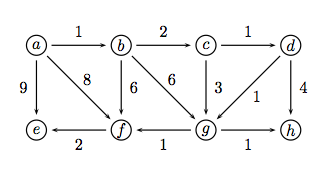# Question Suppose we run Dijkstra's single-source shortest-path algorithm on the weighted directed graph below, starting from node a. When the algorithm terminates, the seven edges (prev[u],u), with u in the set {b,c,d,e,f,g,h}, make up the shortest-path tree. What is the tree's weight, that is, what is the sum of its edges' weights? 2 a с d 8 6 9 6 3 4 e (9 (h 2 1 1S5OSXH The Asker · Computer ScienceSuppose we run Dijkstra's single-source shortest-path algorithm on the weighted directed graph below, starting from node a. When the algorithm terminates, the seven edges (prev[u],u), with u in the set {b,c,d,e,f,g,h}, make up the shortest-path tree.

What is the tree's weight, that is, what is the sum of its edges' weights?

Transcribed Image Text: 2 a с d 8 6 9 6 3 4 e (9 (h 2 1 1
More
Transcribed Image Text: 2 a с d 8 6 9 6 3 4 e (9 (h 2 1 1